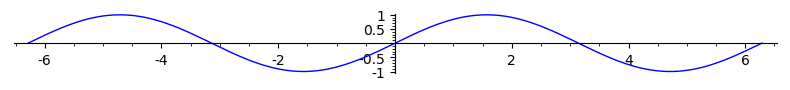# Scaling images

Hi,

I am trying to figure out how to create say an 800 x 600 image that will be able to fit different size sheets of paper. Eventually I would like to expand this algorithm to include all the other screen resolutions. However, when Sage generates an image there is the boarder of white. So I have to go in and crop it out with image editing software. This then changes the pixel dimension. I would like to get a ratio of 1:1 between the m x n image and the image generated. I have figured out this ratio when dpi value is at 100, and it turns out that a dpi value of about 172 will get me really close to creating an image of 800 x 600. However, the actual image is roughly 795 x 596, and if I use 173 it turns out to be 801 x 600.

I am wondering if someone has already created an algorithm to print a 1:1 scale for screen resolution to printed image?

edit retag close merge delete

Sort by » oldest newest most voted

You may do:

sage: p = plot(sin(x), (x, -2*pi, 2*pi))
sage: p.show(figsize=[8,1])


to change the scaling:The method save accepts the same argument:

sage: p.save('image.png', figsize=[8,1])


To do further changes on the figure, you may get the matplotlib object behind the 2d Graphics object and use matplotlib possibilities:

sage: figure = p.matplotlib(figsize=[8,6])
sage: figure
<Figure size 800x600 with 1 Axes>
sage: type(figure)
<class 'matplotlib.figure.Figure'>
sage: axes = figure.axes

more

Thanks for the advise. However, when I tried using figsize that also includes the white border around the image. I am not wanting to include that border. Plus I had to use figsize = [9,7] to get close to 800 x 600, and with using a dpi value of 96. This gave me an image of 795 x 595 after cropping out the white border. I might have to live with this setup since both values are really close to the target values.